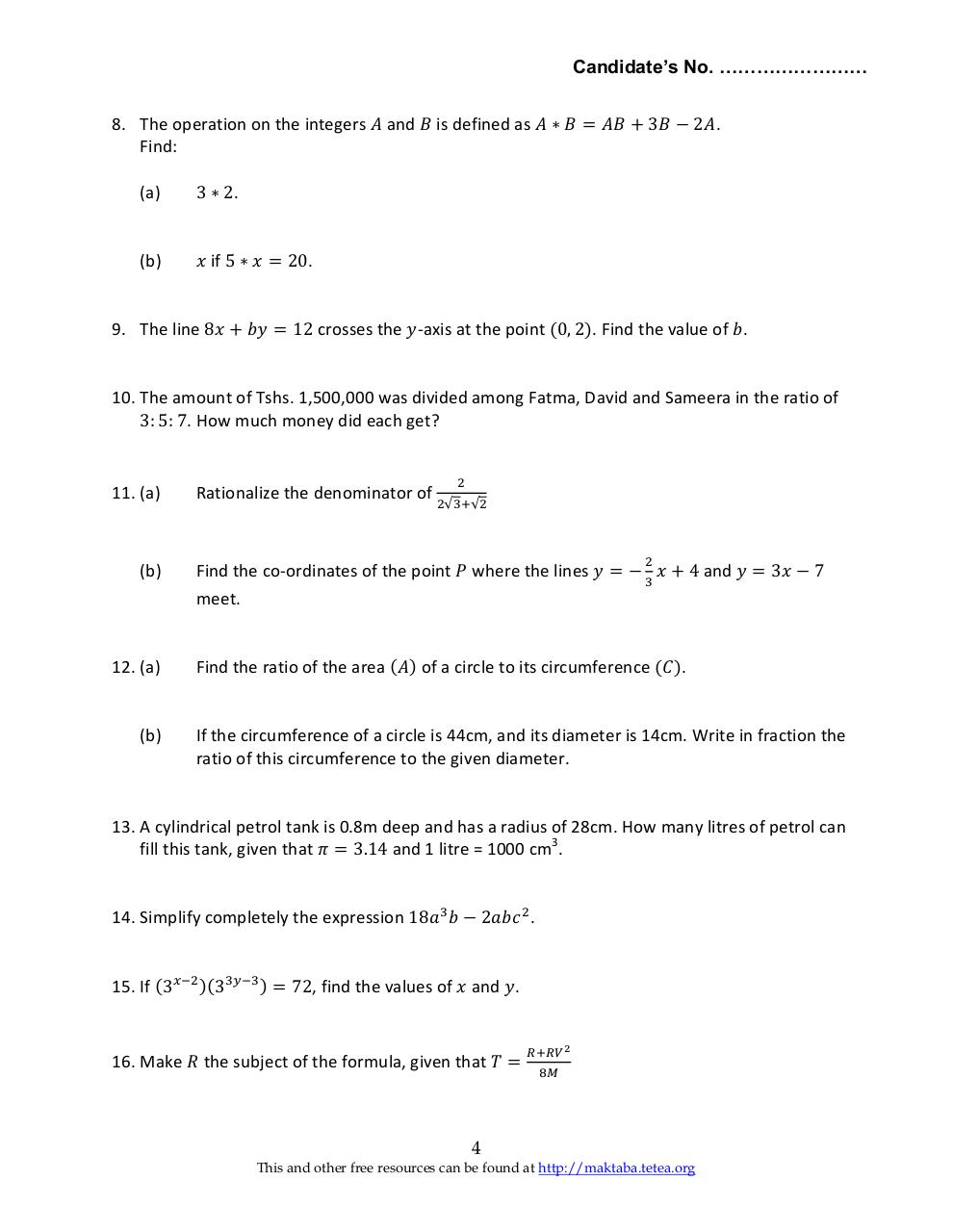# Basic Math 2005 F2.pdfPage 1 2 3 4 5 6

#### Text preview

Candidate’s No. ……………………
8. The operation on the integers 𝐴 and 𝐵 is defined as 𝐴 ∗ 𝐵 = 𝐴𝐵 + 3𝐵 − 2𝐴.
Find:
(a)

3 ∗ 2.

(b)

𝑥 if 5 ∗ 𝑥 = 20.

9. The line 8𝑥 + 𝑏𝑦 = 12 crosses the 𝑦-axis at the point (0, 2). Find the value of 𝑏.

10. The amount of Tshs. 1,500,000 was divided among Fatma, David and Sameera in the ratio of
3: 5: 7. How much money did each get?

11. (a)

(b)

12. (a)

(b)

2

Rationalize the denominator of 2√3+√2
2

Find the co-ordinates of the point 𝑃 where the lines 𝑦 = − 3 𝑥 + 4 and 𝑦 = 3𝑥 − 7
meet.
Find the ratio of the area (𝐴) of a circle to its circumference (𝐶).

If the circumference of a circle is 44cm, and its diameter is 14cm. Write in fraction the
ratio of this circumference to the given diameter.

13. A cylindrical petrol tank is 0.8m deep and has a radius of 28cm. How many litres of petrol can
fill this tank, given that 𝜋 = 3.14 and 1 litre = 1000 cm3.
14. Simplify completely the expression 18𝑎3 𝑏 − 2𝑎𝑏𝑐 2 .
15. If (3𝑥−2 )(33𝑦−3 ) = 72, find the values of 𝑥 and 𝑦.

16. Make 𝑅 the subject of the formula, given that 𝑇 =

𝑅+𝑅𝑉 2
8𝑀

4
This and other free resources can be found at http://maktaba.tetea.org